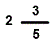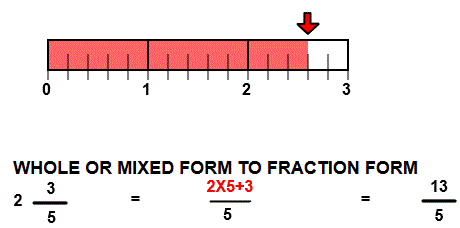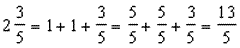# Rename mixed to fraction form

## Number line models picture mixed numbers to fraction(improper) form with numerator and denominator.

MIXED FORM TO FRACTION FORM
CORRECT:
ATTEMPTS:
SCORE:
PERCENT
NUMERATOR
DENOMINATOR
WHOLE OR MIXED FORM
FRACTION FORM

# RENAME MIXED TO FRACTION FORM INSTRUCTIONS

Follow the directions in the dialog box after pressing the <START> button. The <EXPLAIN> button may be pressed to see how to do the example.

Mixed Numbers to Fractions uses number line models to demonstrate how a number in mixed form can be renamed in fraction form.

The illustration below was made by Mixed to Fraction Designer. It shows the mixed number. You are to writein fraction form, with only a numerator and denominator.

In this example, you will notice that the arrow is pointing to the 13th mark after 0, giving a numerator of 13. The number of parts between each whole unit is 5, giving a denominator of 5 for a fraction of 13/5. Some textbooks call a fraction such as this improper, where the numerator is equal to or larger than the denominator. This is also known as the fraction form or a/b form of the number.Arrive at the numerator 13 by multiplying the whole number 2 by the denominator 5 and then add the numerator 3 as in thie above example.

Another way write the example, you can think of each whole unit as 5/5. So in the above example you would have:For more instruction on renaming from mixed form to fraction form go to How to Rename from Mixed form to Fraction Form.

After you enter the fraction form answer you may press the <REPORT> button. The report will ask for your name but you may submit a code for your name. This report will give same results as on the dialog box. The report may be printed or e-mailed.